# Excel formula to count cells with certain text (exact and partial match)

The tutorial shows how to count number of cells with certain text in Excel. You will find formula examples for exact match, partial match and filtered cells.

Last week we looked at how to count cells with text in Excel, meaning all cells with any text. When analyzing large chunks of information, you may also want to know how many cells contain specific text. This tutorial explains how to do it in a simple way.

## How to count cells with specific text in Excel

Microsoft Excel has a special function to conditionally count cells, the COUNTIF function. All you have to do is to supply the target text string in the criteria argument.

Here's a generic Excel formula to count number of cells containing specific text:

COUNTIF(range, "text")

The following example shows it in action. Supposing, you have a list of item IDs in A2:A10 and you want to count the number of cells with a particular id, say "AA-01". Type this string in the second argument, and you will get this simple formula:

`=COUNTIF(A2:A10, "AA-01")`

To enable your users to count cells with any given text without the need to modify the formula, input the text in a predefined cell, say D1, and supply the cell reference:

`=COUNTIF(A2:A10, D1)`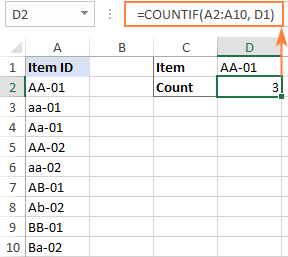Note. The Excel COUNTIF function is case-insensitive, meaning it does not differentiate letter case. To treat uppercase and lowercase characters differently, use this case-sensitive formula.

## How to count cells with certain text (partial match)

The formula discussed in the previous example matches the criteria exactly. If there is at least one different character in a cell, for instance an extra space in the end, that won't be an exact match and such a cell won't be counted.

To find the number of cells that contain certain text as part of their contents, use wildcard characters in your criteria, namely an asterisk (*) that represents any sequence or characters. Depending on your goal, a formula can look like one of the following.

Count cells that contain specific text at the very start:

COUNTIF(range, "text*")

Count cells that contain certain text in any position:

COUNTIF(range, "*text*")

For example, to find how many cells in the range A2:A10 begin with "AA", use this formula:

`=COUNTIF(A2:A10, "AA*")`

To get the count of cells containing "AA" in any position, use this one:

`=COUNTIF(A2:A10, "*AA*")`

To make the formulas more dynamic, replace the hardcoded strings with cell references.

To count cells that begin with certain text:

`=COUNTIF(A2:A10, D1&"*")`

To count cells with certain text anywhere in them:

`=COUNTIF(A2:A10, "*"&D1&"*")`

The screenshot below shows the results: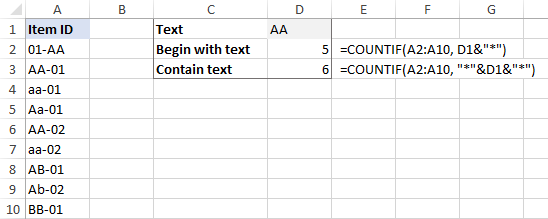## Count cells that contain specific text (case-sensitive)

In situation when you need to differentiate uppercase and lowercase characters, the COUNTIF function won't work. Depending on whether you are looking for an exact or partial match, you will have to build a different formula.

### Case-sensitive formula to count cells with specific text (exact match)

To count the number of cells with certain text recognizing the text case, we will use a combination of the SUMPRODUCT and EXACT functions:

SUMPRODUCT(--EXACT("text", range))

How this formula works:

• EXACT compares each cell in the range against the sample text and returns an array of TRUE and FALSE values, TRUE representing exact matches and FALSE all other cells. A double hyphen (called a double unary) coerces TRUE and FALSE into 1's and 0's.
• SUMPRODUCT sums all the elements of the array. That sum is the number of 1's, which is the number of matches.

For example, to get the number of cells in A2:A10 that contain the text in D1 and handle uppercase and lowercase as different characters, use this formula:

`=SUMPRODUCT(--EXACT(D1, A2:A10))`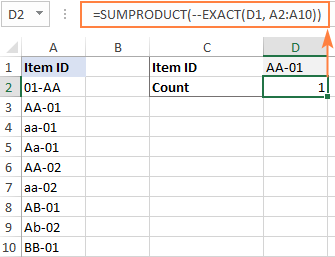### Case-sensitive formula to count cells with specific text (partial match)

To build a case-sensitive formula that can find a text string of interest anywhere in a cell, we are using 3 different functions:

SUMPRODUCT(--(ISNUMBER(FIND("text", range))))

How this formula works:

• The case-sensitive FIND function searches for the target text in each cell of the range. If it succeeds, the function returns the position of the first character, otherwise the #VALUE! error. For the sake of clarity, we do not need to know the exact position, any number (as opposed to error) means that the cell contains the target text.
• The ISNUMBER function handles the array of numbers and errors returned by FIND and converts the numbers to TRUE and anything else to FALSE. A double unary (--) coerces the logical values into ones and zeros.
• SUMPRODUCT sums the array of 1's and 0's and returns the count of cells that contain the specified text as part of their contents.

To test the formula on real-life data, let's find how many cells in A2:A10 contain the substring input in D1:

`=SUMPRODUCT(--(ISNUMBER(FIND(D1, A2:A10))))`

And this returns a count of 3 (cells A2, A3 and A6):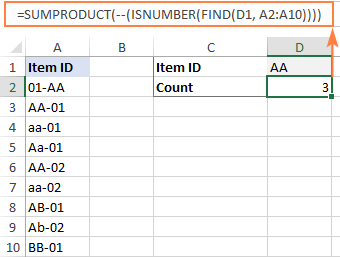## How to count filtered cells with specific text

To count visible items in a filtered list, you will need to use a combination of 4 or more functions depending on whether you want an exact or partial match. To make the examples easier to follow, let's take a quick look at the source data first.

Assuming, you have a table with Order IDs in column B and Quantity in column C like shown in the image below. For the moment, you are interested only in quantities greater than 1 and you filtered your table accordingly. The question is – how do you count filtered cells with a particular id?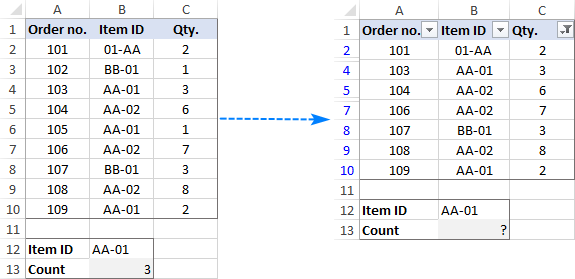### Formula to count filtered cells with specific text (exact match)

To count filtered cells whose contents match the sample text string exactly, use one of the following formulas:

`=SUMPRODUCT(SUBTOTAL(103, INDIRECT("A"&ROW(A2:A10))), --(B2:B10=F1))`

`=SUMPRODUCT(SUBTOTAL(103, OFFSET(A2:A10, ROW(A2:A10) - MIN(ROW(A2:A10)),,1)), --(B2:B10=F1))`

Where F1 is the sample text and B2:B10 are the cells to count.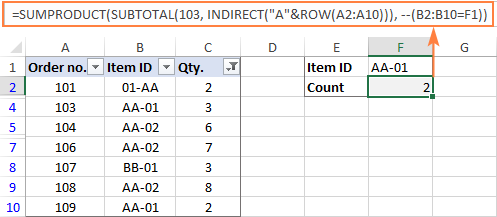How these formulas work:

At the core of both formulas, you perform 2 checks:

1. Identify visible and hidden rows. For this, you use the SUBTOTAL function with the function_num argument set to 103. To supply all the individual cell references to SUBTOTAL, utilize either INDIRECT (in the first formula) or a combination of OFFSET, ROW and MIN (in the second formula). Since we aim to locate visible and hidden rows, it does not really matter which column to reference (A in our example). The result of this operation is an array of 1's and 0's where ones represent visible rows and zeros - hidden rows.
2. Find cells containing given text. For this, compare the sample text (F1) against the range of cells (B2:B10). The result of this operation is an array of TRUE and FALSE values, which are coerced to 1's and 0's with the help of the double unary operator.

Finally, the SUMPRODUCT function multiplies the elements of the two arrays in the same positions, and then sums the resulting array. Because multiplying by zero gives zero, only the cells that have 1 in both arrays have 1 in the final array. The sum of 1's is the number of filtered cells that contain the specified text.

### Formula to count filtered cells with specific text (partial match)

To count filtered cells containing certain text as part of the cell contents, modify the above formulas in the following way. Instead of comparing the sample text against the range of cells, search for the target text by using ISNUMBER and FIND as explained in one of the previous examples:

`=SUMPRODUCT(SUBTOTAL(103, INDIRECT("A"&ROW(A2:A10))), --(ISNUMBER(FIND(F1, B2:B10))))`

`=SUMPRODUCT(SUBTOTAL(103, OFFSET(A2:A10, ROW(A2:A10) - MIN(ROW(A2:A10)),,1)), --(ISNUMBER(FIND(F1, B2:B10))))`

As the result, the formulas will locate a given text string in any position in a cell: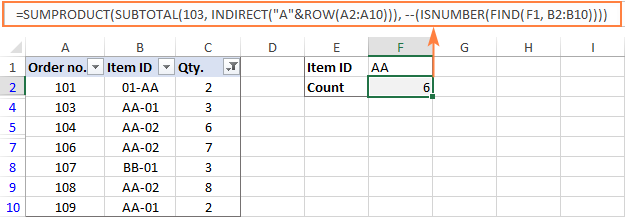Note. The SUBTOTAL function with 103 in the function_num argument, identifies all hidden cells, filtered out and hidden manually. As the result, the above formulas count only visible cells regardless of how invisible cells were hidden. To exclude only filtered out cells but include the ones hidden manually, use 3 for function_num.

That's how to count the number of cells with certain text in Excel. I thank you for reading and hope to see you on our blog next week!

## You may also be interested in

1. Hi sir Alexander, hope you can help me on this one please. We have 2 columns (column A = Names & column B = Status), how can I count the cells with certain names on column A BUT for the status should not have the word DONE.
Example: We want to count the number of cells under the name John but to exclude the word DONE on column B.

• Hi! To count the matches in two columns, use the COUNTIFS function as well as the recommendations from the article above. Here is an example formula:

=COUNTIFS(A1:A10,"John",B1:B10,"<>"&"Done")

2. Hi sir Alexander,

i want to use the same argument to the rest of the items in the column.
=COUNTIF(D:D, "*item name*")
i have 1500 different item names.

How can i automate this argument to the whole column with different item name.

Thank You

• Hi! You can replace the text string in the formula with a cell reference with the item name. For example,

=COUNTIF(D:D,H1)
=SUMPRODUCT(--(D:D=H1))

3. I have a file in which multiple selections are available in a cell. So suppose 4 selections are made in a cell and like that in other cells also multiple selections are made, I want to have a count of individual selections from all the cells.

4. I had this data and the countif function worked:

A2: May 24, 2023 5:05 pm
B2: Lot up

We changed the date (A) to this format: 5/24/2023 17:05

The formula we were using was: =countifs(\$a\$2:\$a\$2000,"may 24, 2023*", \$b\$2:\$b\$2000,"lot up") which worked.
Changing the date to the "5/24/2023*" does not work.

Any suggestions would be greatly appreciated. (I have to use the new date structure)

Thanks,

Bobby

• Hi! I can assume that your date is written as text. So you can only change the format of that date if you change the text. Therefore, the COUNTIFS formula does not work. You can convert text to date in another cell using these guidelines and then apply the date format you want.

5. i want formula for my productivity sheet, i want to calculate columns having % and eliminate holiday columns

6. This is a very informative tutorial. I have tried to create a Power Query DAX measure to do the the same, but I cannot do it. Using the Countif formulae in Excel is much easier. Thanks.

7. Hello!

Hope you can help me with the following.

I have column A with names and B with cost. Different companies sent me their budgets and I want a formula that tells me how much the cost they told me.

Example:

I'm doing a Tab where I put which product and cost per company they budgeted, as not every company offer the same fruits

Company XX (merged columns A and B) Company YY (merged columns C and D)

Column A Column B Column C Column D
Banana 10\$ Melon 15\$
Apple 20\$ Banana 12\$
Melon 15\$

and in another tab I want the totals and say Company XX if they sell bananas, give me the result on the cell next to it, if they don't sell them (if blank) write not bidded. So this new tab will like like

XX YY
Banana 10\$ 12\$
Apple 20\$ not bidded
Melon 15\$ 15\$

I'm trying countif(range:range,banana, BUT I dont know how to say "give me the number in cell next to it and if is blank say "not bidded"

Thank you!!!

8. Hi,
How to count cells with certain numeric value?
For example this is a column A
12
13
21
And I want to find how many "1" counts?
=Countif(a:a,"*1*")
This returns 0 instead of 3
Can you help me
Thanks

• Hi!
The COUNTIF function cannot handle numbers as text. So I recommend searching for and summing partial matches. Here is an example of a formula:

=SUM(--ISNUMBER(SEARCH("1",A1:A15)))

9. Dear Alexander,

I have set up a Google Form linked to a sheet to register visitors to the school where I work.

I'm using conditional formatting with a custom formula "=COUNTIF(B:B,B:B)>1" to apply a rule for instances of names that occur more than once in the column. In this case, it will grey out visitors' names who have completed their visit, leaving only current visitors in regular formatting.

But in the case where a visitor returns for a another visit, their name will appear three (or more times), and therefore will also be greyed out.

How do I modify the formula to only apply to counts that are even numbers, rather than just greater than one?

Thank you!

• Thank you very much, I'll have a chance to plug it in and experiment later ...

10. Hi,
Below is the formula I am using.

=IF(COUNTIF(V4:Y10,"*Issue*"),"PASS","FAIL")

Problem is.... no matter what I do it will give me PASS if its the first option and give me FAIL if I move it to the first option like shown below.

=IF(COUNTIF(V4:Y10,"*Issue*"),"FAIL","PASS")

There is not an "issue" in my range I do not understand why this is not working, but it is on my other sheets.

Anyone able to help me solve this?

Thanks!

• Hi!
I can't check your formula because I don't have your data. I could not repeat your problem.

11. I can't seem to find an answer to a very simple problem: I want to COUNTIF cells whose value does not equal a string, but that string has a space in it.
I have tried the following permutations and they don't work

Any help much appreciated!

• Hi!
If you want to count cells that are not equal to a text string, add the appropriate logical operators to COUNTIFS formula.
For example, try this formula:

12. Looking for a formula that counts specific text in a row. For example in a row of 31 characters how mant times does "V" appear?

13. Good afternoon.

I'm trying to figure out a way to count how many times a certain name appears in a spreadsheet, with a "yes" in the cell next to it.
Is that possible?

Thanks

14. =COUNTIF(MID(\$C\$2:C6,2,4),MID(\$C\$2:C6,2,4))

Hi, is there a way to workaround this error without creating another column to extract the texts first? Need a counter for every unique set of strings in a cell's value without the first and last characters, such that E12231 and N12230 will count as one since "1223" is the same. Is there an alternative to the MID function that can work with ranges?

15. =COUNTIF(MID(\$C\$2:C6,2,4),MID(\$C\$2:C6,2,4))

Hi, is there a way to workaround this error without creating another column to extract the texts first? Need a counter for every unique set of strings in a cell's value without the first and last characters, such that E12231 and N12230 will count as one since "1223" is the same. Is there an alternative to the MID function that can work with ranges?

16. I am attempting to use the COUNTIF but I have issue with names. I have 2 staff; Val and Valentina. I want to count for both but the formula with "Val" is also adding in the "Valentina" information. How do I keep "Val" from adding Valentina's info??? =COUNTIF(O:O,"Val") but it also includes Valentina. How do I add a NOT Valentina to this count cell???

17. Hello, I am looking to count if text contains key words

I need the formula to check for cells that contain "abdo" anywhere in the box and "cramp"

I want this to be able to pick up; crampy abdominal pain, abdominal cramps, abdominal cramping etc

=COUNTIF(G2:G151,"*"&"cramp"&"*"&"*"&"abdo"&"*")

This formula gets only some of the data and I'm not sure how to make it include all of it!

Thanks

• Hello!

=COUNTIF(G2:G151,”cramp”)+COUNTIF(G2:G151,”abdo”)

I'm afraid that returned no results and there are around 12 comments with both pieces of information.

• Excellent! That does indeed work!

=SUMPRODUCT(--ISNUMBER(SEARCH("Small",Sheet1!J2:J145)),--ISNUMBER(SEARCH("bowel",Sheet1!J2:J145)),--ISNUMBER(SEARCH("dist*",Sheet1!J2:J145)*OR("Dila*",Sheet1!J2:J145)))

Here I'm trying to search for the boxes with EITHER small bowel distention OR small bowel dilation.

It does not work, any ideas?

Also, thanks for all your help! It has been, for want of a better word, helpful.

• Hello!
To use the SUMPRODUCT function with OR conditions, use these guidelines: SUMPRODUCT formula with OR logic. Instead of multiplying, use plus symbol (+) between conditions.

18. Hi, this article is linked to the COUNTIF article, in which you can count the number of times items in a list are seen in another list. However, as the article states, COUNTIF is case insensitive, and directs you here for case sensitive.
This article does not provide an alternative method that is case sensitive. Is it possible?

• Hello!
This formula with the SUMPRODUCT functioncounts the number of case-sensitive exact matches in column A and column B

=SUMPRODUCT(--(EXACT(A1:A10,B1:B10)))

19. I am trying to to count only cells that are visible in a sorted list. For example, my current formula is =COUNTIF(\$D\$358:\$D\$95320,A95327). The text in A95327 is EXECUTIVE, however it is counting all EXECUTIVE in the D Column, even the the cells that are hidden due to sorting. Anyone have a solution? Thank you so much.

20. **** UPDATED COMMENT WITH CORRECT FORMULA ******

Hi There,

I am trying to come up with the part of the formula that would allow me to exclude partial match of multiple strings in one. However, the part with "*" does not work for multiple cell selection, as seen in the formula below. Is there alternative efficient way of doing it? The only other way I could think of is to exclude each string individually, but that would be the formula super ugly and long.

Here is the formula:
=SUM(SUMIFS('raw data'!C:C,'raw data'!V:V,"*"&'STEPS - GENERAL'!E27:E30&"*",'raw data'!A:A,"*"&'STEPS - GENERAL'!D36:D57&"*"))

Thank you,

Lilly

• Hi!
It is very difficult to understand a formula that contains unique references to your workbook worksheets.
I assume that the problem is that you are using ranges of different values for the criteria - E27:E30 and D36:D57. From the range D36:D57, only the first 3 values are used: D36, D37, D38. The criteria ranges must be the same size.

• Hi Alexander,

Thanks for a prompt response.

I am not sure why, but the comment removes angles brackets for some reason?

You are right, I should have created a sample formula instead. I'll try again and hopefully the angle brackets will appear this time.

Here is what I am trying to do (sample formula):

Sum the numbers from the column A if the column B contains a partial match of Cells Y1:Y10 and column B does NOT contain partial match of cells Z1:Z30.

I believe the formula should be the following:
=SUM(SUMIFS(A:A,B:B,"*"&Y1:Y10&"*",B:B,"*"&Z1:Z30"*"))

My issue is with the part about does NOT contain as it doesn't. The only way it does work is when I exclude the cells separately =SUM(SUMIFS(A:A,B:B,"*"&Y1:Y10&"*",B:B,"*"&Z1"*",B:B,"*"&Z2"*",B:B,"*"&Z3"*"........))

This way of course the formula becomes super long.

So I was wondering if there is another way of doing the part of does NOT contain partial match for multiple cells in bulk?

Thank you,

Lilija

• Right, so the angle brackets do disappear every time I post a comment. Very weird! The only way is to spell them out in the formula instead then.

My original formula that doesn't work:
=SUM(SUMIFS(A:A,B:B,"*"&Y1:Y10&"*",B:B,"angle brackets*"&Z1:Z30&"*"))

The only way it works is when the cells are excluded separately:
=SUM(SUMIFS(A:A,B:B,"*"&Y1:Y10&"*",B:B,"angle brackets*"&Z1&"*",B:B,"angle brackets*"&Z2&"*",B:B,"angle brackets*"&Z3&"*"........))

I would appreciate any help on this one.

Thank you,

Lilija

• Right, so the angle brackets do disappear when I post a comment. Very weird! I think the only way for me to show the full formula is to spell the angle brackets instead:

The formula that doesn't work is the following:

=SUM(SUMIFS(A:A,B:B,"*"&Y1:Y10&"*",B:B,"angle brackets*"&Z1:Z30&"*"))

I would appreciate any help on this one.

Thank you,

Lilija

21. How can i get something similar to this work? Jack will be on different Classes "A,B,C,D,E,F" and i would like to have a count of how many A classes he has, how many B, C, D, E, F.
formula for each letter will be used for different cells (A on A1, B on B1, C on C1....)

=countifs(X:X=O5,I6,W:W)

Please if anyone has the answer this would be much appreciated. I can do this using a Pivot Table, but i prefer to have the table so it refreshes automatically once a Cell is updated without me refreshing all the time.

Thanks :)

• Thanks Alexander, really helpful - =COUNTIFS(\$A\$2:\$A\$11, "Apples", \$C\$2:\$C\$11,"Cancelled")

22. Hi,
Thank you for the tutorial.
I'm hoping you can help with the following task that I'm trying to achieve.
I have a sales report where it contains sales order number and product code. I'm trying to find out how many order contains a certain set of product only. For example I have product code (A, B, C, D, E) and my data set/sales report are as follows:
Column 1 (Sales Order#) Column2(Product Code)
100 A
100 B
101 E
101 D
101 A
102 B
102 C
103 A
103 E
104 A

I would like to count how many order contains product A, B and/or C only. In the Example above I should have only 3 as the count result (Order# 100, 102 and 104). Order# 101 and 103 are eliminated due to having product code D and E.

23. Hi there,

I want to learn how to create a formula to tally a location from an enquiry. The many examples i've seen the data you want to tally is known, ie how many times is the word apple used. My data is unknown till entered & will change on a weekly basis.

So i have the set cells i want the data tallied from being H7, H13, H19 & H25.

If i use example data that would be inputted into these cells. H7= Liverpool, H13: Fairfield, H19: Oran Park, H25: Liverpool

I want excel to spit out & identify that i had x2 Liverpool, x1 Fairfield & x1 Oran Park.

How is that possible?

24. I can trying to count total instances that match both a specific text value in a column and a second column if ISNUMBERIC. Example data might look like this.

Month | Processed | ID
July | not processed | NULL
July | processed | 123
Aug | not processed | 234
Aug | processed | NULL
Sept | not processed | 765

Here is what I am trying to do in a function and if it worked would return a count of 1 as my result:

The output is in Tab 0 and the data is in Tab 1,

=COUNTIFS('Tab 1'!A:A,"July",'Tab 1'!C:C,ISNUMBER) <-- this doesn't work. I always get zero. ID is number so I know that is not my issue.

So basically looking for July in column A and a numeric value in ID. If that is met then count up the instances.

• Hi!
The argument to the COUNTIFS function cannot be an Excel function. Use the formula

=SUMPRODUCT(--(A1:A12="July"),--(ISNUMBER(C1:C12)))

25. Hello!

I'm trying to total my clinical hours by category. If I have the hours in column B and the category in column D, am I able to use the COUNTIF function to total these hours? So I don't want to count the actual number of cells in column D containing certain text like "aphasia," "language," "articulation," "fluency," but rather locate the columns that have those titles and count the numerical value in column B for the same row?

I don't want to know how many cells contain the word "aphasia" - I want to total the hours I have (column B) in the rows containing "aphasia."

26. Hii,

i have data in cell a1 Like VII,VI,VIII,XI,X,XII,IX

How can i extract Specific Roman text from From Cell A1

27. Hi ,

I would like to calculate a specific text say "ABC" Which present under only particular column name.
Eg: I want to calculate the number of text "ABC" available under the column names "BBB" & "CCC".

• Hi!
Sorry, it's not quite clear what you are trying to achieve. What does the phrase mean "column names “BBB” & “CCC”"?
Pay attention to the following paragraph of the article above — How to count cells with certain text (partial match)

28. Hello,
I am trying to format a document that calculates the different types of days off employees can take at my business. Ideally we would like to format the cells on the calendar with either a V for vacation or S for sick day. Along with the letter, I would like to have the hours used for example if an individual took 8 hours off for a sick day it would read S8. Is there anyway I can write a countif statement where I can separate the days off based on its category and calculate the hours used as well? I tried using a statement you shared with a different user but our situations seem to be too different for it to work for me as well. My end goal is to calculate the hours used each month for the different types of days off so employees can see the hours they have remaining to use. Thank you for any help or insights you may be able to provide me

• Hello!
You didn't say anything about what data you have for calculations.
What columns and what rows does your table have?
However, if you have "S8" written in the cell, then you cannot perform any calculations, since this is text, not a number.

29. Hello,

I'm using the COUNTIF formula however, it does not autocorrect itself to a number once I click enter. The formula still remains when I click away. I have two spreadsheets - it's working fine on one but not the other. I've double checked the formula and it's correct

• Hi,
I think there is a symbol in front of the = sign. Perhaps it is a space or '
Therefore Excel writes your formula as text.

30. Hi. Using COUNTIFS I'm counting specific cells based on 3 criteria. That's working fine however I wish to add 4th criteria of "if a month text in a cell on a line matches a master month cell" on the same sheet, it includes that item in the count. e.g. Feb-21 in a cell on a line and Feb-21 in cell L2, then its included.

31. A1 = Name 123
= COUNTIF(A1,"=*Name #*")

I would like the COUNTIF to be able to count if any number after "Name" is >0. What do I replace # with in the above formula?

• Hello!
Sorry, it's not quite clear what you are trying to achieve. The expression “Name” is > 0 is incorrect because text and number cannot be compared. Could you please describe it in more detail? What result do you want to get? Give an example of the source data and the expected result.

• A1 = Name

B2=COUNTIF(A2,"*"&A\$1&" 0*")
+COUNTIF(A2,"*"&A\$1&" 1*")
+COUNTIF(A2,"*"&A\$1&" 2*")
+COUNTIF(A2,"*"&A\$1&" 3*")
+COUNTIF(A2,"*"&A\$1&" 4*")
+COUNTIF(A2,"*"&A\$1&" 5*")
+COUNTIF(A2,"*"&A\$1&" 6*")
+COUNTIF(A2,"*"&A\$1&" 7*")
+COUNTIF(A2,"*"&A\$1&" 8*")
+COUNTIF(A2,"*"&A\$1&" 9*")

If A2 has "Name" followed by any number (note required space in between), it will count it. For example, it will count "Name 1", "Name 22", or "Name 3A". But it will not count "Name A".

I want to simplify this to one line, and not have to use ten COUNTIF's. However, I'm not sure how to do this. I can't find a logic argument to count cell with "Name" followed by a space and any number.

I need to simplify this because I'm essentially copying this across >50,000 rows in column B to analyze A, and Excel is essentially freezing because the amount of code is so large copying the above across each cell.

• Hello!
If I understand your task correctly, the following formula should work for you:

=SUM(--ISNUMBER( SEARCH(A\$1&" "&{1,2,3,4,5,6,7,8,9,0},A2,1)))

I hope this will help, otherwise please do not hesitate to contact me anytime.

• Yes! Thank you, this works great. I also need to search text across multiple columns. If I extend this through 2 columns in row 2 (i.e., A2:B2), I notice summing it works:

=SUM(--ISNUMBER( SEARCH(A\$1&" "&{1,2,3,4,5,6,7,8,9,0},A2,1))+
--ISNUMBER(SEARCH(A\$1&" "&{1,2,3,4,5,6,7,8,9,0},B2,1)))

But this doesn't work, and I'm not sure why:
=SUM(--ISNUMBER(SEARCH(A\$1&" "&{1,2,3,4,5,6,7,8,9,0},A2:B2,1)))

If I needed to do 20 columns (A2:T2), would I need to take 20 sums, or is there an easier way? For now I only need to do 2 columns of data, but I'm wondering if I could expand it easily to more columns.

• Hello!

=SUM(--ISNUMBER(--MID(\$A\$2:\$T\$2,LEN(\$A\$1)+2,1)))

• Regarding

=SUM(--ISNUMBER(--MID(\$A\$2:\$T\$2,LEN(\$A\$1)+2,1)))

For some reason if B2 is left empty (or does not have "Name" followed by a number), it will result in zero no matter what is in the other 9 cells in row 2.

By the way, I like that this new suggestion does not have to list out numbers 0 to 9 in the code. I do find it strange Excel does not have a wildcard (like * and ?) for a number.

• Hi,
I have not been able to replicate this problem. The formula works if several cells are empty or there is no text "Name"

• Regarding,

=SUM(--ISNUMBER(--MID(\$A\$2:\$T\$2,LEN(\$A\$1)+2,1)))

I found more specifics to the issue.
A1 = Name
A2:T2 contains the data.
Then above equation can't be used in any of the columns A2:T2, otherwise it will result in a zero if the data in that column does not get a hit. I even tried putting equation in another sheet, but same issue occurs. The equation needs to be put outside of columns used for data. Can this be resolved you think?

• For example, put the equation in A3, then you will notice if A2 is empty (or does not have hit of Name #) it will result in a zero no matter what is in the other data cells B2:T2. If you put equation in B3, the same happens for empty cell in B2, and so on.

• Hi,
I did what you wrote. I cannot repeat your problem. My formula is working correctly.

=SUM(--ISNUMBER(--MID(\$A\$2:\$T\$2,LEN(\$A\$1)+2,1)))

I just realized, having studied MID, NUM, ISNUMBER functions, that this will not work because I need to search for specific names followed by a number. In other words, this latest suggestion will not distinguish between "Bob 4" and "Dan 4", because both names have 3 characters. This means we're back to your previous nice suggestion of:

=SUM(--ISNUMBER( SEARCH(A\$1&" "&{1,2,3,4,5,6,7,8,9,0},A2,1)))

which works nice for data in A2, but not if data is in A2:B2 (i.e., several columns in row 2). Do you think there is a work around, other than the summing A2 and B2:

=SUM(--ISNUMBER( SEARCH(A\$1&" "&{1,2,3,4,5,6,7,8,9,0},A2,1))+
--ISNUMBER(SEARCH(A\$1&" "&{1,2,3,4,5,6,7,8,9,0},B2,1)))

• Excel is getting unhappy when I have to copy your equation across many cells

A1 = Name
A2:B100000 contains the data with Name (followed by number) among other random text.
C2 has:

=SUM(--ISNUMBER( SEARCH(A\$1&" "&{1,2,3,4,5,6,7,8,9,0},A2,1))+
--ISNUMBER(SEARCH(A\$1&" "&{1,2,3,4,5,6,7,8,9,0},B2,1)))

which copied down to row 100000.

My goal is to get rid of copying the above equation down 100000 rows, and essentially sum up the 1's and 0's for all 100000 rows of data. Any suggestions on how to calculate each row and then sum them all up without copying the equation down 100000 rows?

32. If I have the following:
A B

1 G 1
2 W 2
3 R 2
4 G 1
5 R 1

Where G, W, R indicates a colour (Green, Red or White) and the numbers in column B indicate what team you are on - what would the formula be to count for example all the R on Team 1?

I can get a formula to count the how many overall are on W, R or G but now I need a formula to work out how many W are on Team 1, How many W are on team 2, How many R are on team 1, how many R are on team 2 etc etc....

Thanks in advance for any help.

• Sorry A is meant to be above G, W, R etc and B above 1, 2, 2 etc for columns - first column 1-5 is the row number.

Thanks

33. Hi, I am trying to find a formula, which can help me count the number "ge-" or "GigabitEthernet' in a cell with these values.

Cell 1 : "ge-1/0/13.0;ge-1/0/14.0;ge-1/0/39.0"

Cell 2 : "GigabitEthernet1/0/2;GigabitEthernet1/0/3;GigabitEthernet1/0/4;"

34. Hi,
I've been trying to solve a problem for a while, but am still struggling.
The task: I have a list of product names (column A), some of which are very similar (e.g. Protos 1, Protos 100, or Tarin 3, Tarin 3 Pro). Cells can contain more than 1 product.
When I use the countif formula with *, I get double counts (every mention of Protos 100 is also counted for Protos 1 etc.).
So I've built the following formula:

=COUNTIFS(K:K,"*Protos 1*",K:K,""*Protos 100*")

However, now I am missing results that mention both: Protos 1 AND Protos 100. So I added another part:

=COUNTIFS(K:K,"*Protos 1*",K:K,""*Protos 100*")+COUNTIFS(K:K,"*Protos 1*",K:K,"*Protos 100*")

The idea for the second Countifs was that I want to validate both criteria: mentions of Protos 1 AND Protos 100. But the problem now is that this part K:K,"*Protos 1*" in the second countif again gives me all of the Protos 100 as well.

Would you have any tip on how to solve this?
Thank you!

• Edit: product names are in column K, not A

• Sorry, saw another copy paste mistake the formulas are:
=COUNTIFS(K:K,"*Protos 1*",K:K,"*Protos 100*")
=COUNTIFS(K:K,"*Protos 1*",K:K,"*Protos 100*")+COUNTIFS(K:K,"*Protos 1*",K:K,"*Protos 100*")

• Hello!
I don't know what separators are used in your values. I assume it's a comma. Then you can use the formula:

=COUNTIF(K:K,"*Protos 1")+COUNTIF(K:K,"*Protos 1,*") + COUNTIF(K:K,"*Protos 100") + COUNTIF(K:K,"*Protos 100,*")

35. Hello! I need to countif the value in c* = .NET AND the value of e* = No. I keep getting value errors and for the life of me can't figure out why. Thanks! This tutorial was very helpful!

• Hello!
Unfortunately, without seeing your data it is difficult to give you any advice. Please provide me with an example of the source data and the expected result. Please specify what formula you used and what problem or error occurred. It’ll help me understand it better and find a solution for you.

36. i want to count the number of cells containing either "*provis*" or "*under*". What should be the formula?

37. Im trying to create a Dashboard on Sheet 1

To count a Specific Word (Column A) based on a Specific Day (Column B) on Sheet 2

38. Hi - I'm looking for a formula which will help me with the following:

To return a value (P for example) in a cell when there is a name within the text of a cell (the text will involve other words than just the name, as it's a description) in a different column that corresponds to a name within a list in a different sheet of the workbook. Multiple names within the list which all need to be checked against what comes up in the cells I'm searching to return a value from.

Any help will be hugely appreciated! Many thanks

• Hello!
It’ll help me understand it better and find a solution for you.

39. Good afternoon,
I have last names in cells in column c. Task is to count all cells in range that contains letter "v" on second place. Would You be so kind and give me solution.

• Hello!
If I understand your task correctly, the following formula should work for you:

=SUM(--(MID(A1:A10,2,1)="v"))

I hope this will help

40. Hi. I have a worksheet with numerous text and numbers. In column A I have different text:A1 AAAA A2: BBBB: A3: AAAA as well. In column be I have numbers B1:1,B2:2,B3:1. How do I lookup for AAAA in column and then count the values which is picked up in column B?

• Hello!
The formula below will do the trick for you:

=SUMPRODUCT(--("AAA"=A1:A15),B1:B15)

• Thanks so much.
I have another problem....
I have also a sheet with multiple texts and 2 sheets.
On sheet 1 I have text AAA in column A
On sheet 2 I have various lines in
Column A Column B Column C
AAA CAT 1
BBB DOG 1
BBB CAT 1
AAA CAT 2
How do I look up only AAA Cat on sheet 2 and count the CAT in various lines so that CAT shows the amount of 3 in sheet 1 for AAA.
Apologies I'm only entry into Excel :-)

• Hello!
To count the number of lines containing both "AAA" and "CAT", use the formula

=SUM(--("AAACAT"=A1:A15&B1:B15))

41. Hello,

I like your tutorials! Very clear and direct. I am trying to sort thru a column containing ship to information. Specifically I am wanting to count the number of orders that were shipped to each state. Sometimes the Stated is spelled out, say Georgia. Other times it is abbreviated, say GA. I can use countif with a wildcard, but then I need create a formula for each state. Is there an easy way to review the range, group it by state and then count the results? Thanks in advance for any help you can provide!

42. Hi
I'm looking for a formula that if column F says 'VA' then the numeric number in the same row but column E needs to be added into a total on a table.
If the cell in column F is changed to a different variable eg 'OS' then the value is subtracted from the 'VA' total and added to the 'OS' total.

• Hello Bianca!
I’m sorry but your task is not entirely clear to me. For me to be able to help you better, please describe your task in more detail. Please specify what you were trying to find, what formula you used and what problem or error occurred. Give an example of the source data and the expected result.
It’ll help me understand it better and find a solution for you. Thank you.

• Bianca, your best bet is to use a pivot table to sumarize data that way. But if you really want it on the same sheet, use SUMIF. E.g. in your case you might have the formula for the total of the VA values have something like =SUMIF(F1:F100,"VA",E1:E100) (assuming you have 100 rows of data)

43. Thank you for your tutorial it was very insightful and helpful to me. I am wondering though if there is a countif formula that will count letters and numbers and then total the numbers? For Example: if I have cells that contain V10, V8, F8, F4, and V2 is there a countif formula that would total the numbers with the V (10+8+2=20)and the F (8+4) and then total the cells so they read V20 or F10?
I do not know if that is even possible but I am hoping that it is.
Thank you very much for your time

• Hello Lori-Ann!
If I understand your task correctly, the following formula should work for you:

=CONCATENATE("V",SUMPRODUCT(--(LEFT(A1:A16,1)="V"), --(IFERROR(RIGHT(A1:A16,LEN(A1:A16)-1),0))))

I hope this will help, otherwise please do not hesitate to contact me anytime.

44. Is there a countif formula to count cells if they do NOT contain specific words. For example, I have a countif formula for everything that contains "BAW" in it. Now I would like a countif formula for everything except "BAW" in it but I need to be able to address multiple words like {"BAW","BAE"} etc. Any help would be appreciated. Thank you.

• Hello Matthew!
If I understand your task correctly, the following formula should work for you:
=SUMPRODUCT(--(\$A\$1:\$A\$17<>\$E\$1),--(\$A\$1:\$A\$17<>\$E\$2))
where in E1 written "BAE", in E2 - "BAW"